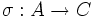# Transitive normality is not finite-join-closed

This article gives the statement, and possibly proof, of a subgroup property (i.e., transitively normal subgroup) not satisfying a subgroup metaproperty (i.e., finite-join-closed subgroup property).This also implies that it does not satisfy the subgroup metaproperty/metaproperties: Strongly finite-join-closed subgroup property (?), .
View all subgroup metaproperty dissatisfactions | View all subgroup metaproperty satisfactions|Get help on looking up metaproperty (dis)satisfactions for subgroup properties
Get more facts about transitively normal subgroup|Get more facts about finite-join-closed subgroup propertyGet more facts about strongly finite-join-closed subgroup property|

## Statement

### Verbal statement

The join of two transitively normal subgroups of a group need not be transitively normal.

### Statement with symbols

Suppose$G$ is a group and$H, K$ are two transitively normal subgroups of$G$. Then, the join$\langle H, K \rangle$, which in this case equals the product$HK$, need not be a transitively normal subgroup.

## Related facts

### Related properties that are join-closed

• Normality is strongly join-closed: In particular, this implies that any join of transitively normal subgroups is a normal subgroup, even though it need not be transitively normal.

## Proof

Further information: symmetric group:S3

Let$A$ be the cyclic group of order three. Let$B$ be the symmetric group of degree three, and$C$ be the subgroup of order three in$B$. Define:$G = A \times B, \qquad H = A \times 1, \qquad K = 1 \times C$.

Then, we have:

•$H$ and$K$ are both normal subgroups of prime order. In particular, the only normal subgroups they have are the whole group and the trivial subgroup, both of which are normal in$G$. Thus,$H$ and$K$ are both transitively normal.
•$HK$ is not transitively normal:$HK = A \times C$.$HK$ is elementary abelian of order nine. Pick an isomorphism$\sigma:A \to C$ and consider the subgroup$L$ of$HK$ given by$L = \{ (a, \sigma(a) \mid a \in A \}$. Then,$L$ is normal in$HK$, since$HK$ is abelian. However,$L$ is not normal in$G$, because conjugation by an element in$1 \times (B \setminus C)$ sends$(a,\sigma(a))$ to$(a,\sigma(a)^2)$, which is not in$L$. Thus,$HK$ is not transitively normal.

Note that in this example,$H$ is the center of$G$ and is also a direct factor of$G$. This shows that taking the join of a transitively normal subgroup with the center or with a direct factor does not guarantee a transitively normal subgroup.